Select Page

In a previous couple of articles, we explored some basic machine learning algorithms and how they fit into the .NET world. Thus far we covered some simple regression algorithms, classification algorithms. Apart from that, we learned a bit about unsupervised learning, more specifically – clustering. We used ML.NET to implement and apply these algorithms. In this article, we explore one of the most popular machine learning algorithms Support Vector Machine or SVM for short.

Are you afraid that AI might take your job? Make sure you are the one who is building it.

STAY RELEVANT IN THE RISING AI INDUSTRY! 🖖

## 1. Dataset and Prerequisites

Data that we use in this article is from PalmerPenguins Dataset. This dataset has been recently introduced as an alternative to the famous Iris dataset. It is created by Dr. Kristen Gorman and the Palmer Station, Antarctica LTER. You can obtain this dataset here, or via Kaggle. This dataset is essentially composed of two datasets, each containing data of 344 penguins. Just like in Iris dataset there are 3 different species of penguins coming from 3 islands in the Palmer Archipelago. Also, these datasets contain culmen dimensions for each species. The culmen is the upper ridge of a bird’s bill. In the simplified penguin’s data, culmen length and depth are renamed as variables culmen_length_mm and culmen_depth_mm.Data itself is not too complicated. In essence, it is just tabular data: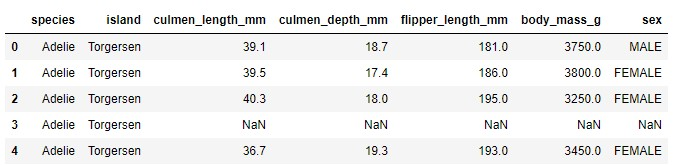Note that in this tutorial, we ignore the species feature. This is because we perform unsupervised learning, ie. we don’t need the expected output value of the sample. We want our algorithm to figure that out on its own. Here is how data looks like when we plot it: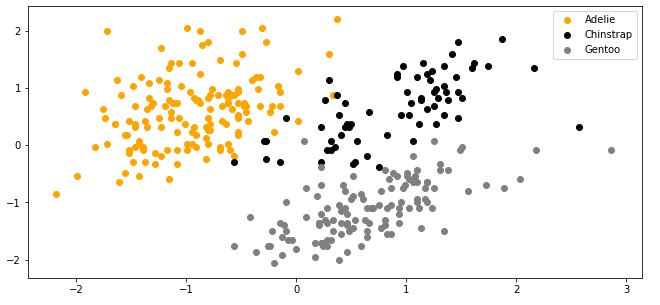The implementations provided here are done in C#, and we use the latest .NET 5. So make sure that you have installed this SDK. If you are using Visual Studio this comes with version 16.8.3. Also, make sure that you have installed the following package:

``Install-Package Microsoft.ML``

You can do a similar thing using Visual Studio’s Manage NuGetPackage option: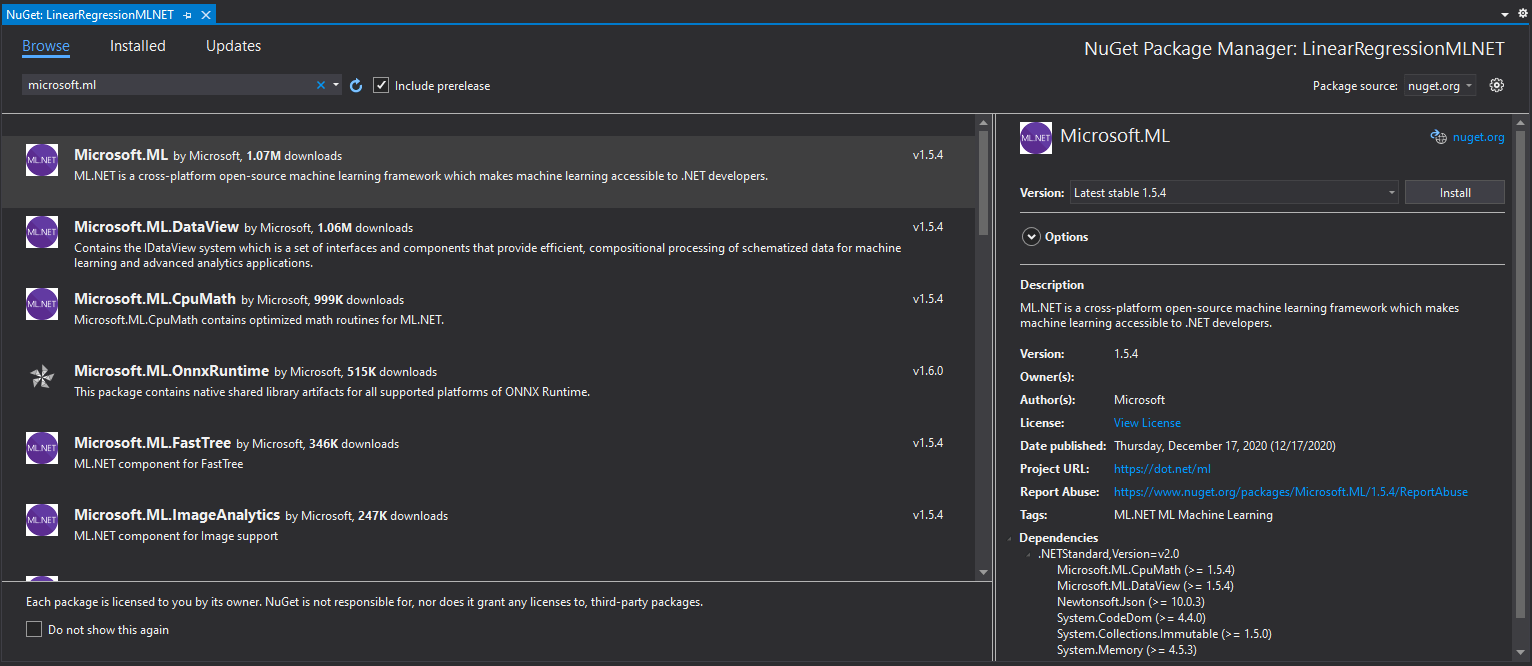If you need to catch up with the basics of machine learning with ML.NET check out this article

## 2. SVM Intuition

Before we implement SVM with ML.NET, we need to learn a bit about this algorithm. SVM is one of the most popular machine learning algorithms and for a good reason. This algorithm proved over and over again to be really good for both – classification and regression and every machine learning engineer should have it in their toolbox. It is also applicable to linear and non-linear data.

### 2.1 SVM for Classification

Let’s first explore how this algorithm works for simple binary classification in order to understand how it functions. This means that we will consider only two classes form the PalmerPenguins dataset. As other machine learning algorithms SVM observers every feature vector as a point in a high-dimensional space. In its core, SVM puts all feature vectors on an imaginary n-dimensional plot and draws an imaginary n-dimensional line (a hyperplane) that separates examples with positive labels from examples with negative labels in the case of classification, or collects as much as samples as possible in case of regression. The hyperplane is defined by the function:where x is the feature vector, w is the feature weights vector with size same as x, and b is the bias term. This is formula should be familiar from our journey through Linear Regression or Logistic Regression. In the case of binary classification, which we consider at the moment, SVM requires that the positive label has a numeric value of 1, and the negative label has a value of -1. This means that the predicted label for some feature vector x can be calculated using the formula: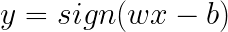The function sign returns value 1 if the input is a positive number and -1 if the input is a negative value. So, SVM model, which during the training process should optimize w and b, can be described with the formula:We can break this down and write it as: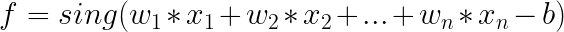This all looks very similar to the Logistic Regression approach. To better understand why this algorithm and what is the difference from Logistic Regression, let’s consider constraints under which the algorithm operates:On a graph that looks like this:This means that SVM doesn’t only create a hyperplane, but it also constructs additional vectors which are defining the margin. These vectors are called support vectors and the distance between the closest examples of two classes is called the margin. Hyperplane with support vectors is often referred to as street. This means that SVM tries to fit the best street between the samples of different classes. Unlike the Logistic regression which tries to fit the hyperplane as close as possible to these points, SVM tries to fit the hyperplane that is as far as possible from the samples but still separates classes successfully.

Note that a large margin leads to a better generalization, meaning the model will better classify new samples. However, notice that the margin is decided by the Euclidean norm of w (denoted by ||w|| in the image). This effectively means the smaller the weight vector w, the larger the margin and thus better generalization. To sum it up, training the SVM algorithm for classification means finding the value of w and b that makes the margin as wide as possible while avoiding misclassification. Meaning, the objective is defined as a constrained optimization problem:where t(i) is –1 for negative samples and t(i) = 1 for positive samples. To be more precise, this optimization problem is a convex quadratic optimization problem with linear constraints. Such problems are known as Quadratic Programming (QP) problems. The solution for this type of problem is outside of the scope of this article. More information in Convex Optimization you can find here and more information for constraint optimization, in general, can be found here

There are many methods to find the optimal w and b for the SVM. One of the most popular ones is Sequential Minimal Optimization (SMO) which is used by the SciKit Learn as well. In its core, the SMO algorithm splits the quadratic programming optimization problem into smaller ones. However, this algorithm is rather complicated so in this article, we implement a simpler one – The Pegasos algorithm. This algorithm uses stochastic gradient descent and it is defined like this: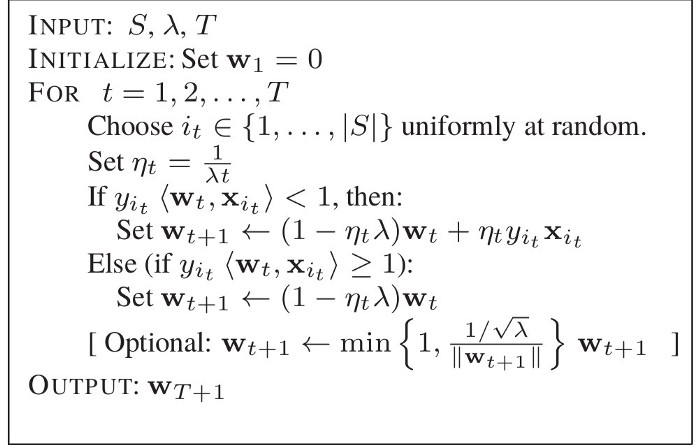### 2.2 Non-Linear Data

Thus far we observed a pretty nice example, data that is linearly separable. In reality, this is almost never the case. So, let’s consider other classes from the PalmerPenguins dataset and load Adelie and Chinstrap classes.This time it is not so easy to separate classes with just a straight line. Data is a bit scrambled, so what should we do in these situations when data is not linear? Here we can apply probably the greatest SVM advantage – kernel trick. This technique gives you the possibility to get the same result as if you were using polynomial features without actually having to add them. Kernels are just functions that map low-dimensional non-linearly-separable data into a linearly-separable high-dimensional data. Meaning, in our case, we map our 2D data which is not linearly separable into 3D data that is.However, we don’t know which mapping works for our data the best, so if we would map all the vectors into a higher dimension and then apply SVM to it that would be very inefficient. That is where the kernel trick comes into play. In essence, it uses kernels to work in higher-dimensional spaces without doing this transformation explicitly. This means that use different kernels on our dataset. For example, we could use the polynomial kernel. However, one of the most popular kernel functions is the Gaussian RBF kernel defined by the formula: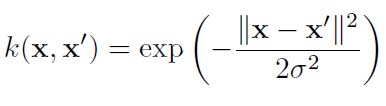It is a bell-shaped function varying from 0 to 1 and it is often used for adding features using similarity features method. Notice the parameter gamma. It is defining how wide the bell-curve is and this essentially the hyperparameter of the SVM. In essence, when we use this kernel we create a gaussian bell-curve in 3-dimensional space, in this example (since our data is in 2-dimensional space), around the chosen landmark.

Then all points are mapped from 2-dimensional space to 3-dimensional space, but every point is mapped to this curve. That is how we ensure that data is linearly separable in 3-dimensional space. Then SVM is applied. Of course kernel trick is applied so we don’t have to do all of these calculations, so the algorithm is pretty efficient. Here is how that would visually look like if we would apply different kernels to our dataset: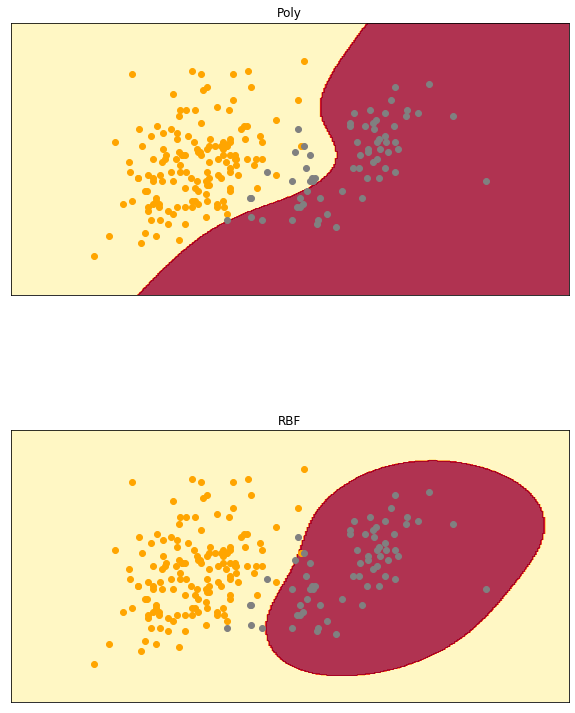### 2.3 SVM for regression

SVM for regression is not so different from the one for the classification. In essence, all the practices that we learned for classification stand for the regression as well, with one major difference. For the classification SVM tried to fit the largest street among the samples of different classes, without violating margins. In regression, SVM tries to fit as many samples as possible on the street, while minimizing number of the samples of the street. The wideness of the street is controlled by hyperparameter – epsilon.

## 3. ML.NET supported SVM Algorithms

Unfortunately, ML.NET support for SVM variations is not too big. Additionally, it is limited only to binary classification. This is quite disappointing and we hope that in the future there will be more support for SVM algorithms. It boils down to two SVM variations, both used only for binary classification:

• Linear SVM – This is ML.NET implementation of the previously mentioned PEGASOS algorithm. Here is how that algorithm works:
1. Input: S, λ, T
2. Initialize: Set w1 = 0
3. For t = 1..T
• Choose it ∈ {1, …, |S|} uniformly at random
• Set nt = 1/λt
• If yit(wt, xit) < 1  then:
• wt+1 ← (1 + nt * λ)wt + nt * yit * xit
• Else
• wt+1 ← (1 + nt * λ)wt
4. Output: wT +1
• Local Deep SVM – This implementation is a generalization of Localized Multiple Kernel Learning for non-linear SVM. In essence, non-linear SVMs are great but can be slow and LD-SVM spreads up this process. As we learned in the previous chapter, non-linear SVM uses kernels, ie. kernel learning. The objective in kernel learning is to jointly learn both kernel and SVM parameters. In particular, Localized Multiple Kernel Learning, which this implementation generalizes, aims to learn a different kernel, and hence classifier, for each point in feature space. This can be time-consuming, especially for large datasets. LD-SVM reduces the prediction cost by learning a tree-based local feature embedding that is high dimensional and sparse, efficiently encoding non-linearities.

## 4. Implementation with ML.NET

As we have learned, ML.NET currently supports only binary classification with SVM. Binary classification is performing simple classification on two classes. In essence, it is used for detecting if some sample represented some event or not. So, simple true-false predictions. That is why we had to modify and pre-process data from PalmerPenguin Dataset. We left two features culmen depth and culmen length. The other features are removed. We also modified the species feature, which now indicated if the sample belongs to the Adelie species or not (1 if the sample represents Adelie; 0 otherwise). Here is how data looks like now:This is a simplified dataset and the problem we want to learn – Does some new sample that comes in our system represents Adelie’s class or not. Here is what that means for our dataset visually:### 4.1 High-Level Architecutre

But first, let’s consider the high-level architecture of this implementation. In general, we want to build an easily extendable solution that we can easily extend with new SVM algorithms that ML.NET will hopefully include in the future. That is why the folder structure of our solution looks like this: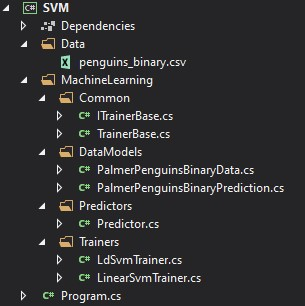The Data folder contains .csv with input data and the MachineLearning folder contains everything that is necessary for our algorithm to work. The architectural overview can be represented like this: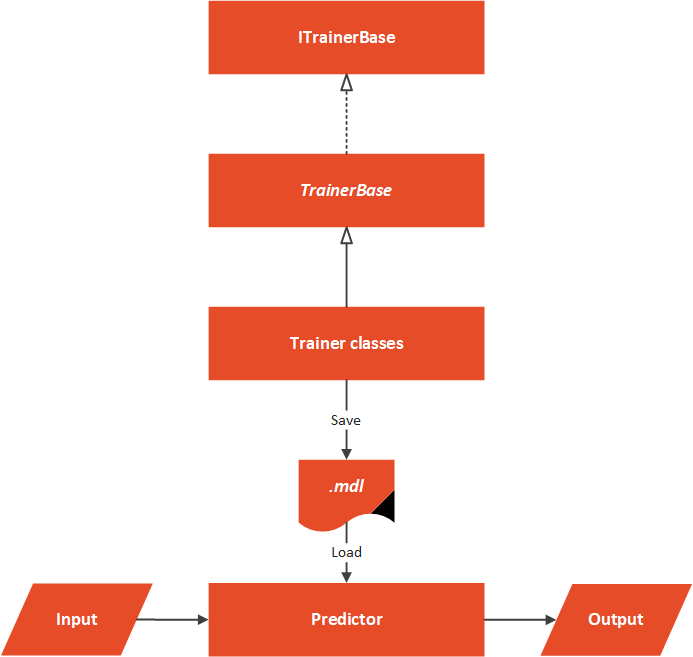At the core of this solution, we have an abstract TrainerBase class. This class is in the Common folder and its main goal is to standardize the way this whole process is done. It is in this class where we process data and perform feature engineering. This class is also in charge of training machine learning algorithm. The classes that implement this abstract class are located in the Trainers folder. Here we can find multiple classes which utilize ML.NET algorithms. These classes define which algorithm should be used. In this particular case, we have only one Predictor located in the Predictor folder.

### 4.2 Data Models

In order to load data from the dataset and use it with ML.NET algorithms, we need to implement classes that are going to model this data. Two files can be found in Data Folder: PalmerPenguinBinaryData and PricePalmerPenguinBinaryPredictions. The PalmerPenguinBinaryData class models input data and it looks like this:

``````using Microsoft.ML.Data;

namespace SVM.MachineLearning.DataModels
{
/// <summary>
/// Models Palmer Penguins Binary Data.
/// </summary>
public class PalmerPenguinsBinaryData
{
public bool Label { get; set; }

public float CulmenLength { get; set; }

public float CulmenDepth { get; set; }
}
}
``````The PricePalmerPenguinBinaryPredictions class models output data:

``````using Microsoft.ML.Data;

namespace SVM.MachineLearning.DataModels
{
/// <summary>
/// Models Palmer Penguins Binary Prediction.
/// </summary>
public class PalmerPenguinsBinaryPrediction
{
public bool PredictedLabel { get; set; }
}
}
``````

### 4.3 TrainerBase and ITrainerBase

As we mentioned, this class is the core of this implementation. In essence, there are two parts to it. The first one is the interface that describes this class and another is the abstract class that needs to be overridden with the concrete implementations, however, it implements interface methods. Here is the ITrainerBase interface:

``````using Microsoft.ML.Data;

namespace SVM.MachineLearning.Common
{
public interface ITrainerBase
{
string Name { get; }
void Fit(string trainingFileName);
BinaryClassificationMetrics Evaluate();
void Save();
}
}``````

The TrainerBase class implements this interface. However, it is abstract since we want to inject specific algorithms:

``````using SVM.MachineLearning.DataModels;
using Microsoft.ML;
using Microsoft.ML.Calibrators;
using Microsoft.ML.Data;
using Microsoft.ML.Trainers;
using Microsoft.ML.Transforms;
using System;
using System.IO;

namespace SVM.MachineLearning.Common

{
/// <summary>
/// Base class for Trainers.
/// This class exposes methods for training, evaluating and saving ML Models.
/// Classes that inherit this class need to assing concrete model and name.
/// </summary>
public abstract class TrainerBase<TParameters> : ITrainerBase
where TParameters : class
{
public string Name { get; protected set; }

protected static string ModelPath => Path
.Combine(AppContext.BaseDirectory, "svm.mdl");

protected DataOperationsCatalog.TrainTestData _dataSplit;
protected ITrainerEstimator<BinaryPredictionTransformer<TParameters>, TParameters> _model;
protected ITransformer _trainedModel;

protected TrainerBase()
{
MlContext = new MLContext(111);
}

/// <summary>
/// Train model on defined data.
/// </summary>
/// <param name="trainingFileName"></param>
public void Fit(string trainingFileName)
{
if (!File.Exists(trainingFileName))
{
throw new FileNotFoundException(\$"File {trainingFileName} doesn't exist.");
}

var dataProcessPipeline = BuildDataProcessingPipeline();
var trainingPipeline = dataProcessPipeline.Append(_model);

_trainedModel = trainingPipeline.Fit(_dataSplit.TrainSet);
}

/// <summary>
/// Evaluate trained model.
/// </summary>
/// <returns>Model performance.</returns>
public BinaryClassificationMetrics Evaluate()
{
var testSetTransform = _trainedModel.Transform(_dataSplit.TestSet);

return MlContext.BinaryClassification.EvaluateNonCalibrated(testSetTransform);
}

/// <summary>
/// Save Model in the file.
/// </summary>
public void Save()
{
MlContext.Model.Save(_trainedModel, _dataSplit.TrainSet.Schema, ModelPath);
}

/// <summary>
/// Feature engeneering and data pre-processing.
/// </summary>
/// <returns>Data Processing Pipeline.</returns>
private EstimatorChain<NormalizingTransformer> BuildDataProcessingPipeline()
{
var dataProcessPipeline = MlContext.Transforms.Concatenate("Features",
nameof(PalmerPenguinsBinaryData.CulmenDepth),
nameof(PalmerPenguinsBinaryData.CulmenLength)
)
.Append(MlContext.Transforms.NormalizeMinMax("Features", "Features"))
.AppendCacheCheckpoint(MlContext);

return dataProcessPipeline;
}

{
var trainingDataView = MlContext.Data
return MlContext.Data.TrainTestSplit(trainingDataView, testFraction: 0.3);
}
}
}
``````That is one large class. It controls the whole process. Let’s split it up and see what it is all about. First, let’s observe the fields and properties of this class:

``````public string Name { get; protected set; }

protected static string ModelPath => Path.Combine(AppContext.BaseDirectory, "svm.mdl");

protected DataOperationsCatalog.TrainTestData _dataSplit;
protected ITrainerEstimator<BinaryPredictionTransformer<TParameters>, TParameters> _model;
protected ITransformer _trainedModel;``````

The Name property is used by the class that inherits this one to add the name of the algorithm. The ModelPath field is there to define where we will store our model once it is trained. Note that the file name has .mdl extension. Then we have our MlContext so we can use ML.NET functionalities. Don’t forget that this class is a singleton, so there will be only one in our solution. The _dataSplit field contains loaded data. Data is split into train and test datasets within this structure.

The field _model is used by the child classes. These classes define which machine learning algorithm is used in this field. The _trainedModel field is the resulting model that should be evaluated and saved. In essence, the only job of the class that inherits and implements this one is to define the algorithm that should be used, by instantiating an object of the desired algorithm as _model

Cool, let’s now explore Fit() method:

``````public void Fit(string trainingFileName)
{
if (!File.Exists(trainingFileName))
{
throw new FileNotFoundException(\$"File {trainingFileName} doesn't exist.");
}

var dataProcessPipeline = BuildDataProcessingPipeline();
var trainingPipeline = dataProcessPipeline.Append(_model);

_trainedModel = trainingPipeline.Fit(_dataSplit.TrainSet);
}``````

This method is the blueprint for the training of the algorithms. As an input parameter, it receives the path to the .csv file. After we confirm that the file exists we use the private method LoadAndPrepareData. This method loads data into memory and splits it into two datasets, train and test dataset. We store the returning value into _dataSplit because we need a test dataset for the evaluation phase. Then we call BuildDataProcessingPipeline().This is the method that performs data pre-processing and feature engineering. For this data, there is no need for some heavy work, we just do the normalization. Here is the method:

``````private EstimatorChain<NormalizingTransformer> BuildDataProcessingPipeline()
{
var dataProcessPipeline = MlContext.Transforms.Concatenate("Features",
nameof(PalmerPenguinsBinaryData.CulmenDepth),
nameof(PalmerPenguinsBinaryData.CulmenLength)
)
.Append(MlContext.Transforms.NormalizeMinMax("Features", "Features"))
.AppendCacheCheckpoint(MlContext);

return dataProcessPipeline;
}``````

Next is the Evaluate() method:

``````public RegressionMetrics Evaluate()
{
var testSetTransform = _trainedModel.Transform(_dataSplit.TestSet);

return MlContext.Regression.Evaluate(testSetTransform);
}``````

It is a pretty simple method that creates a Transformer object by using _trainedModel and test Dataset. Then we utilize MlContext to retrieve regression metrics. Finally, let’s check out Save() method:

``````public void Save()
{
MlContext.Model.Save(_trainedModel, _dataSplit.TrainSet.Schema, ModelPath);
}``````

This is another simple method that just uses MLContext to save the model into the defined path.

### 4.4 Trainers

Thanks to all the heavy lifting that we have done in the TrainerBase class, the other Trainer classes are pretty simple and focused only on instantiating the ML.NET algorithm. We have two classes that utilize ML.NET‘s binary SVM classifiers. Let’ take a look at LinearSVMTrainer class:

``````using Microsoft.ML;
using Microsoft.ML.Trainers;
using SVM.MachineLearning.Common;

namespace SVM.MachineLearning.Trainers
{
public class LinearSvmTrainer : TrainerBase<LinearBinaryModelParameters>
{
public LinearSvmTrainer() : base()
{
Name = "Linear SVM";
_model = MlContext.BinaryClassification.Trainers.LinearSvm(labelColumnName: "Label");
}
}
}
``````

As you can see, we only replace Name and create an instance of ML.NET’s LinearSvm class. The class that implements LD-SVM is a bit different:

``````using Microsoft.ML;
using Microsoft.ML.Trainers;
using SVM.MachineLearning.Common;

namespace SVM.MachineLearning.Trainers
{
public class LdSvmTrainer : TrainerBase<LdSvmModelParameters>
{
public LdSvmTrainer(int treeDepth) : base()
{
Name = \$"LD-SVM with {treeDepth} tree depth";
_model = MlContext.BinaryClassification.Trainers.LdSvm(labelColumnName: "Label",
treeDepth: treeDepth);
}
}
}
``````

Apart from giving the specific name and creating an object of LdSvm class, in this class, we utilize the treeDepth hyperparameters. It is injected through the constructor and used to create an LdSvm instance.

### 4.5 Predictor

The Predictor class is here to load the saved model and run some predictions. Usually, this class is not a part of the same microservice as trainers. We usually have one microservice that is performing the training of the model. This model is saved into file, from which the other model loads it and run predictions based on the user input. Here is how this class looks like:

``````public class Predictor
{
protected static string ModelPath => Path.Combine(AppContext.BaseDirectory, "svm.mdl");

private ITransformer _model;

public Predictor()
{
_mlContext = new MLContext(111);
}

/// <summary>
/// Runs prediction on new data.
/// </summary>
/// <param name="newSample">New data sample.</param>
/// <returns>An object which contains predictions made by model.</returns>
public PalmerPenguinsBinaryPrediction Predict(PalmerPenguinsBinaryData newSample)
{

var predictionEngine = _mlContext.Model.
CreatePredictionEngine<PalmerPenguinsBinaryData, PalmerPenguinsBinaryPrediction>(_model);

return predictionEngine.Predict(newSample);
}

{
if (!File.Exists(ModelPath))
{
throw new FileNotFoundException(\$"File {ModelPath} doesn't exist.");
}

using (var stream = new FileStream(ModelPath,
FileMode.Open,
{
}

if (_model == null)
{
throw new Exception(\$"Failed to load Model");
}
}
}``````

In a nutshell, the model is loaded from a defined file, and predictions are made on the new sample. Note that we need to create PredictionEngine to do so.### 4.6 Usage and Results

Ok, let’s put all of this together.

``````using SVM.MachineLearning.Common;
using SVM.MachineLearning.DataModels;
using SVM.MachineLearning.Predictors;
using SVM.MachineLearning.Trainers;
using System;
using System.Collections.Generic;

namespace SVM
{
class Program
{

static void Main(string[] args)
{
var newSample = new PalmerPenguinsBinaryData
{
CulmenDepth = 1.2f,
CulmenLength = 1.1f
};

var trainers = new List<ITrainerBase>
{
new LdSvmTrainer(3),
new LdSvmTrainer(5),
new LdSvmTrainer(7),
new LinearSvmTrainer()
};

trainers.ForEach(t => TrainEvaluatePredict(t, newSample));
}

static void TrainEvaluatePredict(ITrainerBase trainer, PalmerPenguinsBinaryData newSample)
{
Console.WriteLine("*******************************");
Console.WriteLine(\$"{ trainer.Name }");
Console.WriteLine("*******************************");

trainer.Fit("C:\\Users\\n.zivkovic\\source\\repos\\LogisticRegressionFromScratch\\BinaryClassificationMLNET\\Data\\penguins_binary.csv");

var modelMetrics = trainer.Evaluate();

Console.WriteLine(\$"Accuracy: {modelMetrics.Accuracy:0.##}{Environment.NewLine}" +
\$"F1 Score: {modelMetrics.F1Score:#.##}{Environment.NewLine}" +
\$"Positive Precision: {modelMetrics.PositivePrecision:#.##}{Environment.NewLine}" +
\$"Negative Precision: {modelMetrics.NegativePrecision:0.##}{Environment.NewLine}" +
\$"Positive Recall: {modelMetrics.PositiveRecall:#.##}{Environment.NewLine}" +
\$"Negative Recall: {modelMetrics.NegativeRecall:#.##}{Environment.NewLine}" +
\$"Area Under Precision Recall Curve: {modelMetrics.AreaUnderPrecisionRecallCurve:#.##}{Environment.NewLine}");

trainer.Save();

var predictor = new Predictor();
var prediction = predictor.Predict(newSample);
Console.WriteLine("------------------------------");
Console.WriteLine(\$"Prediction: {prediction.PredictedLabel:#.##}");
Console.WriteLine("------------------------------");
}
}
}
``````

Not the TrainEvaluatePredict() method. This method does the heavy lifting here. In this method, we can inject an instance of the class that inherits TrainerBase and a new sample that we want to be predicted. Then we call Fit() method to train the algorithm. Then we call Evaluate() method and print out the metrics. Finally, we save the model. Once that is done, we create an instance of Predictor, call Predict() method with a new sample and print out the predictions. In the Main, we create a list of trainer objects, and then we call TrainEvaluatePredict on these objects. Here are the results:

``````*******************************
LD-SVM with 3 tree depth
*******************************
Accuracy: 0.97
F1 Score: .97
Positive Precision: .96
Negative Precision: 0.98
Positive Recall: .98
Negative Recall: .96
Area Under Precision Recall Curve: 1

------------------------------
Prediction: True
------------------------------
*******************************
LD-SVM with 5 tree depth
*******************************
Accuracy: 0.98
F1 Score: .98
Positive Precision: .98
Negative Precision: 0.98
Positive Recall: .98
Negative Recall: .98
Area Under Precision Recall Curve: 1

------------------------------
Prediction: True
------------------------------
*******************************
LD-SVM with 7 tree depth
*******************************
Accuracy: 0.97
F1 Score: .97
Positive Precision: .96
Negative Precision: 0.98
Positive Recall: .98
Negative Recall: .96
Area Under Precision Recall Curve: 1

------------------------------
Prediction: True
------------------------------
*******************************
Linear SVM
*******************************
Accuracy: 0.95
F1 Score: .95
Positive Precision: .92
Negative Precision: 0.98
Positive Recall: .98
Negative Recall: .93
Area Under Precision Recall Curve: 1

------------------------------
Prediction: True
------------------------------``````

Awesome, so we got different predictions from different algorithms, along with different metrics. All versions gave the correct answer since for the sample we provided we used one of the Adelie instances. Metrics give us the feeling that the LD-SVM algorithm with a tree depth of 5 performed the best. This should be of course taken with a grain of salt and further test the model.

## Conclusion

In this article, we covered a large topic of SVM. We had a chance to see how it can handle both, classification and for regression. We also saw how we can handle linear data and how we can handle non-linear data using kernel trick. We used ML.NET to implement it for Palmer Penguin classification. SVM is one amazing algorithm that is an important part of your Machine Learning toolbox.#### Nikola M. Zivkovic

CAIO at Rubik's Code

Nikola M. Zivkovic a CAIO at Rubik’s Code and the author of book “Deep Learning for Programmers“. He is loves knowledge sharing, and he is experienced speaker. You can find him speaking at meetups, conferences and as a guest lecturer at the University of Novi Sad.

Rubik’s Code is a boutique data science and software service company with more than 10 years of experience in Machine Learning, Artificial Intelligence & Software development. Check out the services we provide.

Ultimate Guide to Machine Learning with Python

Everything from Python basics to the deployment of Machine Learning algorithms to production in one place.

Become a Machine Learning Superhero TODAY!